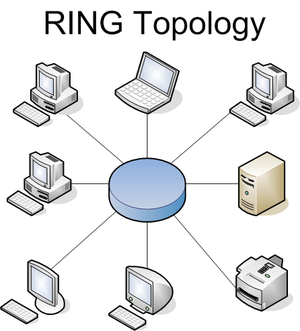Types Of Network TopologiesEnglish: This is a diagram of Ring Topology in Computer Network (Photo credit: Wikipedia)

The design structure of links of computer nodes is defined as network topology. It could also be characterized as the way in which the elements of a network are mapped or arranged. Network topology basically depicts the configuration of a communication network. Here the term “Configuration” means the the physical and the logical interconnection between the different nodes of a network.

Basic categories of network topology
Network topology could be categorized into 3 types,namely – Physical,Logical and Signal topologies.  Physical  topology describes the physical design of a network. Signal topology traces the paths of the signals over the network. The mapping of the paths taken by data as they move over the network is defined as a logical topology. Signal topology and logical topology are almost similar.

Fundamental network topology types
There are 7 fundamental network topology types,viz.
1. Bus topology
2. Star topology
3. Ring topology
4. Tree topology
5. Mesh topology
6. Hybrid topology
7. Point-to-point topology

Bus Topology
All elements of the Bus topology network are linked to a common transmission medium with two end points. All signals, that move over the network, are transmitted through a common transmission medium, called bus.

Star topology
In Star topology network, all nodes are linked to a central node, called hub. The signals that travel back and forth between the nodes, always pass through the hub.

Ring topology
Every point in the Ring topology network is linked to 2 other points and the first and last points are linked to each other.

Tree topology
In Tree topology  network, there is a central node, that is linked to one or more nodes of a lower hierarchy.

Mesh topology
Each network element is connected to every other element in the Mesh topology network.

Hybrid topology
A hybrid topology network is a combination of two or more basic network topologies, such as a star-bus, star-ring, or tiered topology.

Point-to-point topology
In point to point topology network there is a permanent link between two nodes. Conventional telephony is an example of this topology.